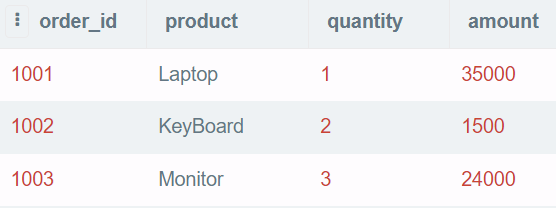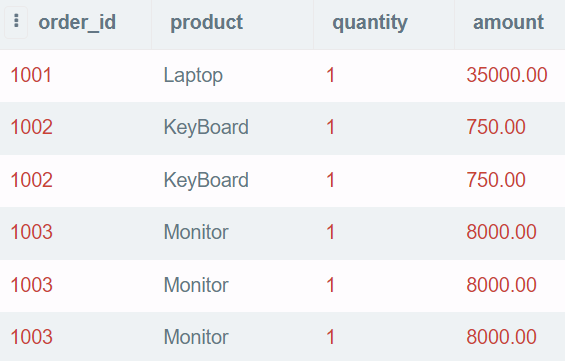# Problem Statement:-

You have an orders table with order details. You have to ungroup the data based on the order quantity.

The amount column in the output should be an amount of a single quantity. Assume that the product's amount will be the same regardless of the quantity ordered.

Write an SQL query to ungroup the orders table.

## Sample Input:-## Expected Output:-## SQL Scripts:-

CREATE TABLE order_details(
order_id INT,
product VARCHAR(255),
quantity INT,
amount INT
);

INSERT INTO order_detail (order_id,product,quantity,amount) VALUES
(1001,'Laptop',1,35000),
(1002,'KeyBoard',2,1500),
(1003,'Monitor',3,24000);

## My Solution:-

I have implemented the solution using Number Sequence and CROSS JOIN. First I have Generated the Number Sequence using RECURSIVE CTE and then CROSS JOIN this Number Sequence table with the Orders table based on the quantity in the Orders table.

## Final Query:-

DECLARE @max_num int = (SELECT max(quantity) FROM order_details);
;WITH numbers (num) as
( SELECT 1 as num

UNION ALL

SELECT num+1
FROM numbers
WHERE num< @max_num
)

SELECT order_id, product,
1 AS quantity, CAST(amount / quantity as DECIMAL(18,2)) AS amount
FROM order_details
CROSS JOIN  numbers
WHERE quantity>= num
ORDER BY order_id, product;

Thanks for reading. I hope you enjoyed this fun little case study - it was fun for me to create!. You can also share your approach to solve this problem.